Example for versions Sanscript 2.2

Sanscript is a fully visual programming language, so no source code is available. See screeshots instead.

This example contains a lot of branches on condition, and in Sanscript each branch is described in a new flowgram.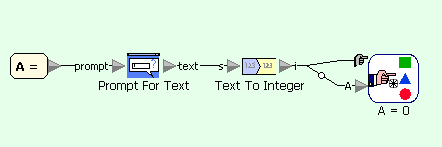Quadratic equation example in Sanscript (main flowgram)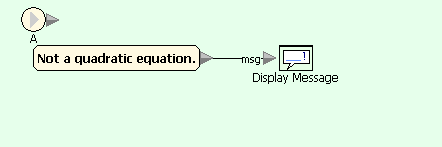Quadratic equation example in Sanscript (on condition A=0)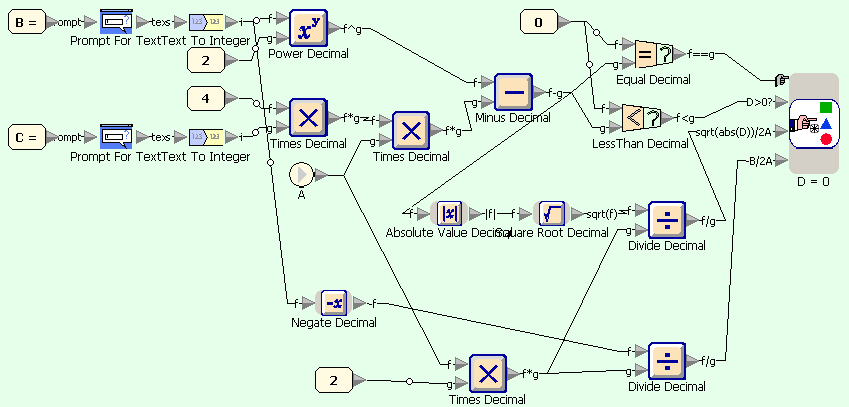Quadratic equation example in Sanscript (on condition A=default)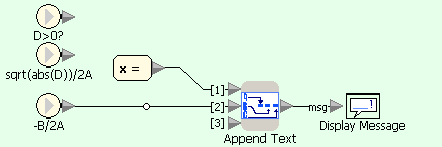Quadratic equation example in Sanscript (on condition D=0)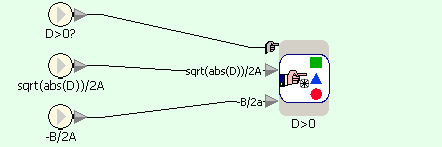Quadratic equation example in Sanscript (on condition D=default)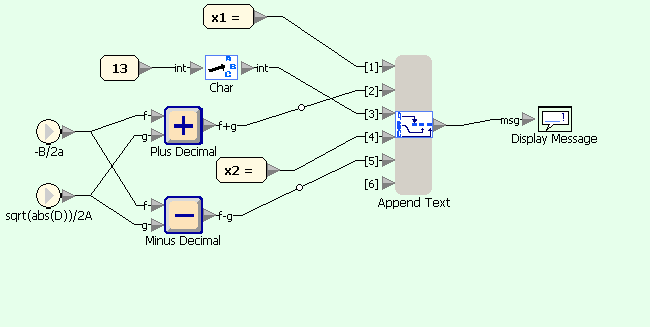Quadratic equation example in Sanscript (on condition D>0)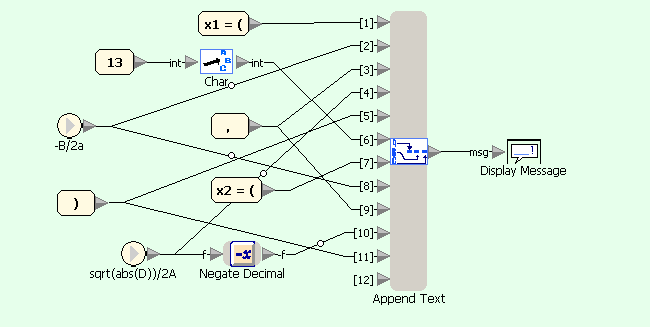Quadratic equation example in Sanscript (on condition D<0)